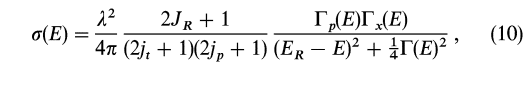# Breit-Wigner formula derivation

• Stickybees

#### Stickybees

Does anyone know where this forumula comes from?http://iopscience.iop.org/0067-0049/134/1/151/fulltext/

I don't quite understand the resonance widths, it's probably a naive question but what is 'resonating' in this context?

Thanks!

I know nothing about this material. However, looking at the formula, it looks like E is resonating around ER.

Much like electron orbitals, the nucleons (protons and neutrons) in an atom have discrete energy states. When you have an interaction between an nucleus and an incident particle (proton or neutron for example), the probability that the incident particle will be absorbed is greatly increased if its energy resonates with the energy state of the product (or intermediate) nucleus. The Breit-Wigner formula describes this interaction for certain types of resonances. These resonances have a finite width in energy.

## 1. What is the Breit-Wigner formula and what does it describe?

The Breit-Wigner formula is a mathematical equation used in particle physics to describe the probability of a particle decaying into a specific set of final states. It takes into account the energy dependence of the decay and is commonly used to study resonances in particle interactions.

## 2. How is the Breit-Wigner formula derived?

The Breit-Wigner formula is derived from the principles of quantum mechanics and the concept of a particle's wave function. It involves integrating over the energy of the particle, taking into account the energy and width of the resonance and the couplings of the initial and final states.

## 3. What are the assumptions made in the derivation of the Breit-Wigner formula?

The derivation of the Breit-Wigner formula relies on several assumptions, including that the particle in question is a stable and isolated resonance, that it has a well-defined mass and lifetime, and that the interactions between the initial and final states are dominated by a single resonance.

## 4. How accurate is the Breit-Wigner formula in describing particle decays?

The Breit-Wigner formula is a simplified model and therefore may not accurately describe all aspects of particle decays. It is most useful for describing narrow resonances and may not be applicable for broader or more complex resonant structures. Experimental data is often needed to confirm the accuracy of the formula for a particular decay process.

## 5. Can the Breit-Wigner formula be used for all types of particles and interactions?

The Breit-Wigner formula is most commonly used in the study of particles and interactions in the realm of high energy physics. It is applicable to a wide range of particle types, including mesons, baryons, and virtual particles. However, it may not be suitable for describing all possible interactions and further studies may be needed to validate its use in specific cases.